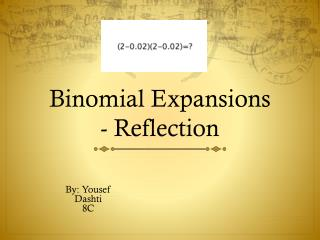DownloadDownload PresentationBinomial Expansions - Reflection

# Binomial Expansions - Reflection

Télécharger la présentation## Binomial Expansions - Reflection

- - - - - - - - - - - - - - - - - - - - - - - - - - - E N D - - - - - - - - - - - - - - - - - - - - - - - - - - -
##### Presentation Transcript

1. Binomial Expansions- Reflection By: Yousef Dashti 8C

2. In this unit we have learned about Binomial Expansions, where we did an investigation referring to this method: Example: (0.99)² =(1-0.01)(1-0.01) =1²-2x1x0.01+0.01² I will refer to it in my reflection as Binomial Expansion method.

3. If I was an engineer 100 years ago…

4. I would…use Binomial Expansion method rather than long multiplication. Since the numbers that we choose in this method are incredibly simple to multiply. Seeing that Long multiplication is hard, it will waste my time and will be very confusing. Example: 59² = (60-1)(60-1) = 360-60-60+1 = 241

5. I wouldn’t use very big numbers since it will make us think twice when using it in the Binomial expansion method. I would mainly say that it would be tricky for a number having three digits or more. It is hard to actually multiply huge numbers like 26,038 or 3.548. Therefore if you use long multiplication it will make the working out much easier. So for example 1.382²=1382 x 1382= 1,909,924. So I place the decimal point 6 places from the right. Therefore the answer will be 1.909924 .

6. I would use Long multiplication because it would be more efficient if we were trying to find a decimal number squared. Since it would be difficult if we tried it with Binomial Expansion. It would be hard to multiply 0.418 by itself using the Binomial Expansion. So that is why I prefer the Long multiplication method: First I add the number of digits in both numbers so for example 0.93 x 0.93 = 4 digits after the decimal. Then I multiply it without the decimal points, so it will be 93 x 93 = 8649. So then I add the decimal point 4 places from the right, which will equal to 0.8649 .

7. In conclusion I would say that using Long multiplication is much easier than using the Binomial Expansion method. As I gave many explanations and examples of how it is not complicated. I would encourage everyone to use Long Multiplication for anything they need to multiply.

8. Thank You For Paying Attention